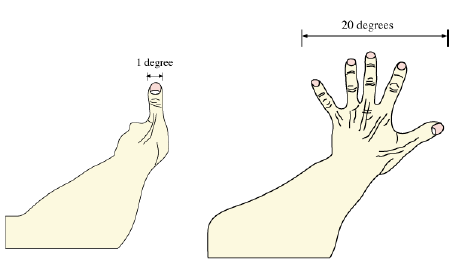# Units

Updated 2019-09-04

## Units

Units are used to standardize quantitative measurements. In this course we mostly use mks (meter, kilogram, seconds) system although cgs (centimeter, gram, seconds) is also common in astronomy as it makes Maxwell’s equations simpler.

### Light-Year

Light-year is a derived unit which describes a distance a beam of light travels in one year. The duration of a year is derived from the Julian year which is exactly 365¼ days. Light speed “C”, is a physical constant, and is exactly 2.99792×108 m/s. Therefore, a light year is exactly: \begin{aligned} ly&=C\times365.25{\text{d}}\times24{\text{h/d}}\times60{\text{m/h}}\times 60 {\text{s/m}}\\ &=(2.99792\times10^8{\text{m/s}})\times(3.15576\times 10^7{\text{s}})\\ &=9.46072\times10^{15}\text{m} \end{aligned}

### Degrees, Arcminute, and Arcsecond

We can estimate angle in our field of view by extending our arms straight out and using the fingers as reference.In a full revolution of a 2D circle, there are 360 degrees. In each single degree, there are 60 arcminutes. In each arcminute, there are 60 arcseconds.